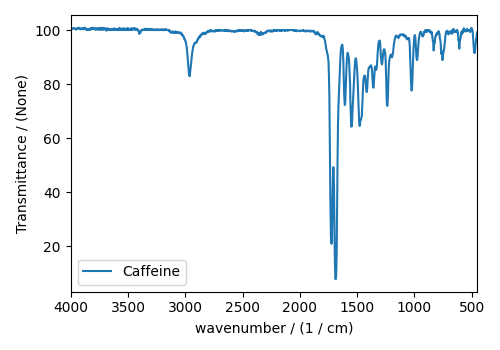Fourier Transform Infrared Spectroscopy (FTIR) dataset¶

The following FTIR dataset, was obtained as a JCAMP-DX file, and subsequently converted to the CSD model file-format. The data structure of the FTIR dataset follows,

import matplotlib.pyplot as plt

import csdmpy as cp

filename = "https://osu.box.com/shared/static/0iw0egupb1hkulkbdq4hagzzhkbvqjkv.csdf"
print(FTIR_data.data_structure)

Out:

{
"csdm": {
"version": "1.0",
"timestamp": "2019-07-01T21:03:42Z",
"description": "An IR spectrum of caffeine.",
"dimensions": [
{
"type": "linear",
"count": 1842,
"increment": "1.930548614883216 cm^-1",
"coordinates_offset": "449.41 cm^-1",
"quantity_name": "wavenumber",
"reciprocal": {
"quantity_name": "length"
}
}
],
"dependent_variables": [
{
"type": "internal",
"name": "Caffeine",
"numeric_type": "float32",
"quantity_type": "scalar",
"component_labels": [
"Transmittance"
],
"components": [
[
"99.31053, 99.08212, ..., 100.22944, 100.22944"
]
]
}
]
}
}

and the corresponding plot.

plt.figure(figsize=(5, 3.5))
ax = plt.subplot(projection="csdm")
ax.plot(FTIR_data)
ax.invert_xaxis()
plt.tight_layout()
plt.show()Because, FTIR spectrum is conventionally displayed on a reverse axis, an optional reverse_axis argument is provided to the plot() method. Its value is an order list of boolean, corresponding to the order of the dimensions.

Total running time of the script: ( 0 minutes 1.296 seconds)

Gallery generated by Sphinx-Gallery